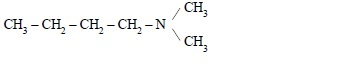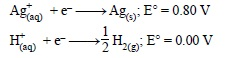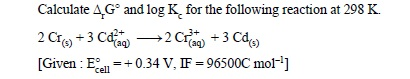# CBSE Class-12 Question Papers for IOP/Comptt Examination 2017 : All India Scheme, Chemistry

Disclaimer: This website is not at associated with CBSE, For official website of CBSE visit - www.cbse.nic.in

## All India Scheme, (Chemistry)

### CBSE Class-12 Question Papers for IOP/Cmptt Examination :  Chemistry (Set-1)

CHEMISTRY (Theory)

Time allowed : 3 hours

Maximum Marks : 70

General Instructions :
(i) All questions are compulsory.
(ii) Question number 1 to 5 are very short-answer questions and carry 1 mark each.
(iii) Question number 6 to 10 are short-answer questions and carry 2 marks each.
(iv) Question number 11 to 22 are also short-answer questions and carry 3 marks each.
(v) Question number 23 is a value based question and carry 4 marks.
(vi) Question number 24 to 26 are long-answer questions and carry 5 marks each.
(vii) Use log tables, if necessary. Use of calculators is not allowed.

1. What type of stoichiometric defect is shown by ZnS ?

2. What are emulsions ? Give one example.

3.Write IUPAC name of the complex : [CoCl2(en)2]+.

4. Write IUPAC name of the following compound :6. Following reactions can occur at cathode during the electrolysis of aqueous silver nitrate solution using Pt electrodes :On the basis of their standard electrode potential values, which reaction is feasible at cathode and why ?

7. “Orthophosphoric acid (H3PO4) is not a reducing agent whereas hypophosphorus acid (H3PO2) is a strong reducing agent.” Explain and justify the above statement with the help of a suitable example.

8. (a) Explain why H2 and O2 do not react at room temperature.
(b) Write the rate equation for the reaction A2 + 3B2 ––> 2C, if the overall order of the reaction is zero.
OR
Derive integrated rate equation for rate constant of a first order reaction.

9.Explain the following observations :
(i) Copper atom has completely filled d orbitals (3d10) in its ground state, yet it is regarded as a transition element.
(ii) Cr2+ is a stronger reducing agent than Fe2+ in aqueous solutions.

10.How will you carry out the following conversions :
(i) 2-Bromopropane to 1-bromopropane
(ii) Benzene to p-chloronitrobenzene

11.An element exists in bcc lattice with a cell edge of 288 pm. Calculate its molar mass if its density is 7.2 g/cm3.

12.13. For a first order reaction, show that time required for 99% completion is twice the time required for completion of 90% reaction.

14. Explain the following phenomenon giving reasons :
(i) Tyndall effect
(ii) Brownian movement
(iii) Physical adsorption decreases with increase in temperature.